Courses

# Introduction: The Force Method of Analysis - 1 Civil Engineering (CE) Notes | EduRev

## Civil Engineering (CE) : Introduction: The Force Method of Analysis - 1 Civil Engineering (CE) Notes | EduRev

The document Introduction: The Force Method of Analysis - 1 Civil Engineering (CE) Notes | EduRev is a part of the Civil Engineering (CE) Course Structural Analysis.
All you need of Civil Engineering (CE) at this link: Civil Engineering (CE)

Since twentieth century, indeterminate structures are being widely used for its obvious merits. It may be recalled that, in the case of indeterminate structures either the reactions or the internal forces cannot be determined from equations of statics alone. In such structures, the number of reactions or the number of internal forces exceeds the number of static equilibrium equations. In addition to equilibrium equations, compatibility equations are used to evaluate the unknown reactions and internal forces in statically indeterminate structure. In the analysis of indeterminate structure it is necessary to satisfy the equilibrium equations (implying that the structure is in equilibrium) compatibility equations (requirement if for assuring the continuity of the structure without any breaks) and force displacement equations (the way in which displacement are related to forces). We have two distinct method of analysis for statically indeterminate structure depending upon how the above equations are satisfied:

1. Force method of analysis (also known as flexibility method of analysis, method of consistent deformation, flexibility matrix method)
2. Displacement method of analysis (also known as stiffness matrix method). In the force method of analysis, primary unknown are forces. In this method compatibility equations are written for displacement and rotations (which are calculated by force displacement equations). Solving these equations, redundant forces are calculated. Once the redundant forces are calculated, the remaining reactions are evaluated by equations of equilibrium.

In the displacement method of analysis, the primary unknowns are the displacements. In this method, first force -displacement relations are computed and subsequently equations are written satisfying the equilibrium conditions of the structure. After determining the unknown displacements, the other forces are calculated satisfying the compatibility conditions and force displacement relations. The displacement-based method is amenable to computer programming and hence the method is being widely used in the modern day structural analysis.
In general, the maximum deflection and the maximum stresses are small as compared to statically determinate structure. For example, consider two beams of identical cross section and span carrying uniformly distributed load as shown in Fig. 7.1a and Fig. 7.1b.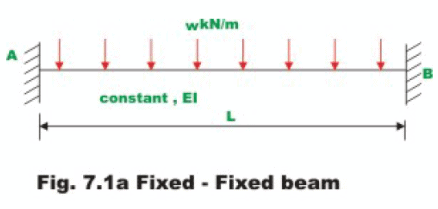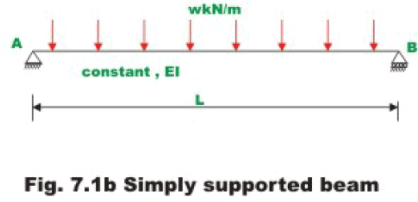The loads are also the same in both cases. In the first case, the beam is fixed at both ends and thus is statically indeterminate. The simply supported beam in Fig. 7.1b is a statically determinate structure. The maximum bending moment in case of fixed- fixed beam is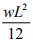(which occurs at the supports) as compared to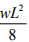(at the centre) in case of simply supported beam. Also in the present case, the deflection in the case of fixed- fixed beam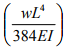is five times smaller than that of simply supported beam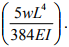Also, there is redistribution of stresses in the case of redundant structure. Hence if one member fails, structure does not collapse suddenly. The remaining members carry the load. The determinate structural system collapses if one member fails. However, there are disadvantages in using indeterminate structures. Due to support settlement, there will be additional stresses in the case of redundant structures where as determinate structures are not affected by support settlement. The analysis of indeterminate structure differs mainly in two aspects as compared to determinate structure.

a) To evaluate stresses in indeterminate structures, apart from sectional properties (area of cross section and moment of inertia), elastic properties are also required.
b) Stresses are developed in indeterminate structure due to support settlements, temperature change and fabrication errors etc.

Instructional Objectives

After reading this chapter the student will be

1. Able to analyse statically indeterminate structure of degree one.
2. Able to solve the problem by either treating reaction or moment as redundant.
3. Able to draw shear force and bending moment diagram for statically indeterminate beams.
4. Able to state advantages and limitations of force method of analysis.

Introduction.

In this lesson, a general introduction is given to the force method of analysis of indeterminate structure is given. In the next lesson, this method would be applied to statically indeterminate beams. Initially the method is introduced with the help of a simple problem and subsequently it is discussed in detail. The flexibility method of analysis or force method of analysis (or method of consistent deformation) was originally developed by J. Maxwell in 1864 and O. C. Mohr in 1874. Since flexibility method requires deflection of statically determinate structure, a table of formulas for deflections for various load cases and boundary conditions is also given in this lesson for ready use. The force method of analysis is not convenient for computer programming as the choice of redundant is not unique. Further, the bandwidth of the flexibility matrix in the force method is much larger than the stiffness method. However it is very useful for hand computation.

Simple Example

Consider a propped cantilever beam (of constant flexural rigidity EI, and span L), which is carrying uniformly distributed load of w kN/m., as shown in Fig. 7.2a. The beam is statically indeterminate i.e. its reaction cannot be evaluated from equations of statics alone. To solve the above problem by force method proceeds as follows.

1) Determine the degree of statical indeterminacy. In the present case it is one. Identify the reaction, which can be treated as redundant in the analysis. In the present case RB or MA can be treated as redundant. Selecting RB as as the redundant, the procedure is illustrated. Subsequently, it will be shown how to attack the problem by treating MA as redundant.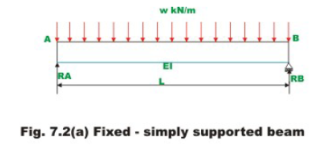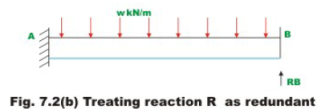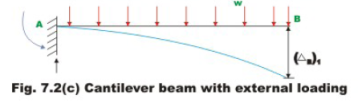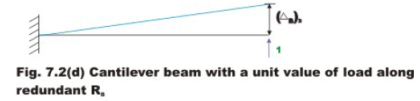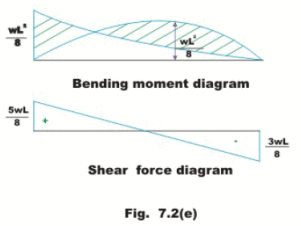Offer running on EduRev: Apply code STAYHOME200 to get INR 200 off on our premium plan EduRev Infinity!

## Structural Analysis

30 videos|122 docs|28 tests

,

,

,

,

,

,

,

,

,

,

,

,

,

,

,

,

,

,

,

,

,

;